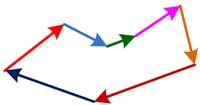## Vector Addition

Two or more vectors of the same dimension (i.e. the same number of elements) can be added together to produce a resultant vector that is also at the same size as the input vectors.

Algebraically, summation of two vectors is done by adding each element of the vectors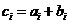.

Example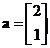,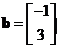,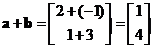Geometrically, summation of two vectors is performed by joining the final point of the first vector to the initial point of the second vector. As a vector can be displaced to a parallel line to the line of application (i.e. a line containing the vector), we can also create parallelogram to produce the resultant vector as shown in the figure below.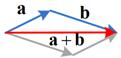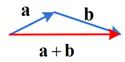The interactive program below show you the algebraic part of the vector addition. Your input must be two vectors of the same dimension (one vector for each text box) and the program will produce the result of vector addition.

+

## Properties

Some important properties of vector addition are

• Vector addition is a commutative operation. You can reverse the order of addition and still get the same result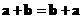• Vector addition is an associative operation. Exchanging the parentheses (to say which order to be computed first) does not change the result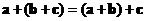.
• Zero vector is a unique additive identity vector such that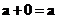. Geometrically, summation of vectors that produce zero-vector will for a closed polygon.